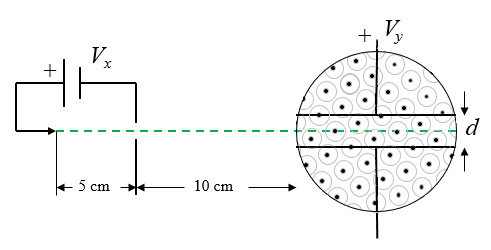Numerical Methods

## J. J. Thomson's experiment to determine the charge-to-mass ratio of electrons

J. J. Thomson performed experiments to show that atoms consisted of sub atomic particles that had positive and negative charges. He determined that the negatively charged particles (electrons) were much lighter than the positively charged particles. The electron charge-to-mass ratio was measured by accelerating the electrons through a voltage $V_x$ towards a positively charged plate. Some of the electrons pass through a small hole in the plate and form and electron beam that travels to a region where an electric field and a magnetic field was present. The electrons were accelerated in the $x$-direction, the electric field was in the $y$-direction and the magnetic field was in the $z$-direction.The force on an electron due to the electric field is $\vec{F}_{\text{elec}}=-|e|E_y\hat{y}$ and the force on an electron due to the magnetic field is $\vec{F}_{\text{magn}}=|e|v_xB\hat{y}$. The velocity of the electrons can be determined because as the electrons are accelerated in the $x$-direction, the potential energy $|e|V_x$ is converted into kinetic energy, $\frac{1}{2}mv_x^2=|e|V_x$. If the experiment is adjusted so that the electric and magnetic forces cancel each other out $|e|v_xB\hat{y}-|e|E_y\hat{y}=0$, then the charge-to-mass ratio is given by, in the $y$-direction Therefore $v_x=\sqrt{2|e|V_x/m}$ and the charge-to-mass ratio is,

$\begin{equation} \frac{e}{m}=\frac{V_y^2}{2V_x\mu_0^2n^2I^2d^2}. \end{equation}$

The simulation below uses metal plates 10  long with a spacing $d=$ 2 cm. The solenoid that generates the magnetic field has a diameter of 10 cm.

 $V_x=$ 5000 [V] $Vy=$ 1 [V] $I=$ 1 [A] $n=$ 2000 [turns/m] $B=$  T $y=$  m $\frac{V_y^2}{2V_x\mu_0^2n^2I^2d^2}=$  C/kg Try to minimize the $y$-value after the electrons have passed through the region with the fields. The accepted value of $\frac{e}{m}$ is $1.7588\times10^{11}$ C/kg. The numerical integration is not perfect.

### Numerical 6th order differential equation solver

 $\large \frac{dx}{dt}=$ $v_x$ $\large \frac{dv_x}{dt}=$ -0.1*vx-sin(x)+sin(3*t) $\large \frac{dy}{dt}=$ $v_y$ $\large \frac{dv_y}{dt}=$ -y $\large \frac{dz}{dt}=$ $v_z$ $\large \frac{dv_z}{dt}=$ 3-0.01*vz Initial conditions: $t_0=$ $\Delta t=$ $x(t_0)=$ $N_{steps}$ $v_x(t_0)=$ Plot: t x vx y vy z vz vs. t x vx y vy z vz $y(t_0)=$ $v_y(t_0)=$ $z(t_0)=$ $v_z(t_0)=$

 $t$   $x$   $v_x$   $y$   $v_y$   $z$   $v_z$